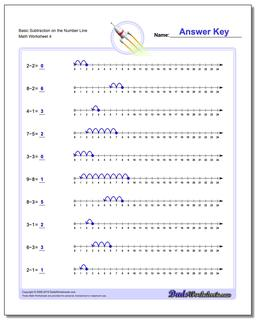# Math Worksheets: Subtraction: Subtraction: Basic Subtraction on the Number Line (Fourth Worksheet)## Basic Subtraction on the Number Line (Fourth Worksheet)

PropertyValue
DescriptionBasic Subtraction on the Number Line: These simple subtraction worksheets introduce subtraction concepts using the number Line. (Fourth Worksheet)
Resource TypeWorksheet